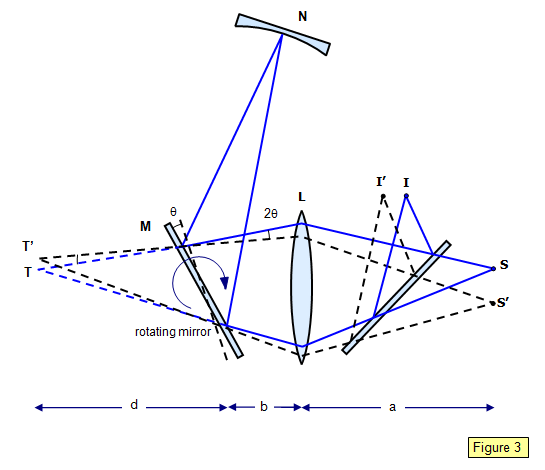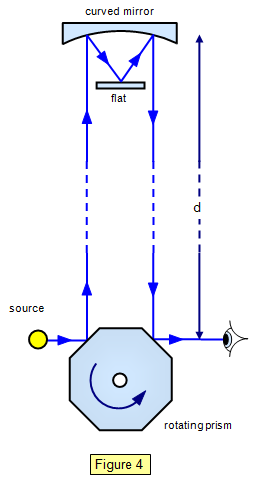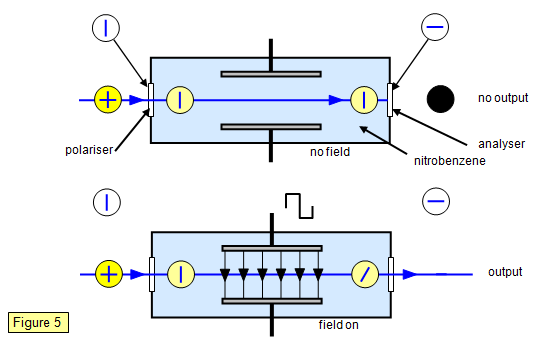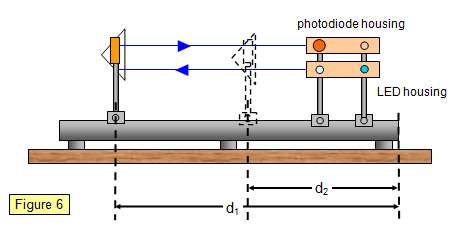# The speed of light

The problem of the nature of light puzzled scientists for many centuries, and it was not until the sixteenth century that some definite ideas were proposed.
Descartes (1596-1650) believed that light was propagated in a similar way to pressure, that is, instantaneously. In 1679, however, Römer proposed that light did have a finite speed and by measuring the time intervals between successive eclipses of the moons of Jupiter was able to measure this speed. He found that the times measured were not the same as those predicted on the basis of an infinite velocity of light. From his observations he predicted that the speed of light was about 190 000 miles per second, or some 3.11x108 ms- 1.

In 1905 Einstein showed that the velocity of light was a fundamental constant, in fact the 'ultimate' velocity for any object.

## Measuring the speed of light

Communication with radio or light waves is limited by the velocity with which the waves can travel. For instance, signals sent to the deep space probe Pioneer 10 take five hours at present to go from the Earth to the spacecraft, so that course corrections have to be sent at least five hours before they are required!
The knowledge of the value of the speed of light is important in the measurement of the light year in astronomy.
The main problem with the measurement of the speed of light is its very large value. We therefore have to use very large distances or very accurate timing methods. Most of the methods to be described here rely on chopping up the light into short pulses; the method of doing this varies from one experiment to another.

## Fizeau's rotating wheel

This was the first terrestrial method for measuring the velocity of light and was performed in 1849.

Light from a source S is focused by a lens L1 on to the edge of a toothed wheel W which has N teeth. It passes through the gaps between the teeth and on to a second lens L2, which produces a beam of parallel light. This travels across a known distance d to a third lens L3 and on to a concave mirror M. It is then reflected back and when it meets plate P it is reflected into the eyepiece E (Figure 1).The wheel is now rotated until a speed is reached at which the pulse of light leaving through one gap returns to the wheel when the next tooth has taken the place of the gap (Figure 2). No light will therefore be observed reaching the eyepiece. If the wheel is rotating at n revolutions per second then

Distance travelled by light = 2d
Transit time = 1/2nN
Speed of light (c) = 4nNd

Fizeau's values were:
2d = 17.26 km, N = 720, n = 12.6 revs per second, giving a value of 3.13 x 108 ms-1 for the speed of light.

The light must be monochromatic to give a parallel beam and the teeth on the wheel should be blackened to stop unwanted reflections.

## Foucault's rotating mirror

First performed in 1862, this experiment can be carried out in the laboratory and is the basis of commercially produced apparatus which used to be available for schools. Light from the source S is converged by a lens L towards a plane mirror M. After reflection the light travels to a concave mirror N and then reflects back along its original path to M and thence into the eyepiece forming an image at I. The plane mirror is now rotated at angular velocity w. During the time the light was travelling from M to N and back again the plane mirror will have rotated through a small angle 0 and so the light reflected from M will be turned through 20 and therefore the image seen in the eyepiece will have moved from I to I' (Figure 3).Let the distance from M to N be d and let the number of revolutions per second of M be n.

q= wt = wx2d/c = 2pnx2d/c = 4πnd/c
But TT' = 2θd and TT'/(d + b) = II'/a
Therefore: z = II'(d + b)/2ad

Therefore the velocity of light (c) is given by the equation:

Speed of light (c) = 8pnd2a/II’(d + b)

Foucault used a mirror with a radius of curvature of 20 m and with a rate of rotation of 800 revolutions per second. II' was 0.7 mm.
M and N could be placed in water so that Foucault was able to measure the velocity of light in a liquid. He showed that light travelled slower in water than it did in air, in fact:

speed of light in air = refractive index of water x speed of light in water

## Michelson's rotating prism

Michelson used a rapidly rotating prism to chop up the light beam in an experiment first carried out in 1879.He mounted the prism on Mount Wilson in the USA and placed a reflector on Mount San Antonio, 35 km away. Light from the source S was reflected from one face of the prism and onto the distant reflector. When the light returned the prism had rotated, and if light was to be observed at the eyepiece it must have rotated by one-eighth of a turn (Figure 4).
One facet of the prism must have replaced its predecessor in the time it takes the light to travel to the distant reflector and back. If d is the distance to the distant reflector and n the number of revolutions per second then:

Speed of light (c) = 16nd

Michelson rotated the prism with an air jet at 528 revs s-1 and measured the frequency with an electrically driven tuning fork that was compared stroboscopically with the rotating prism. He obtained a value for the speed of light of 2.99910 x 108 ± 50ms-1. This value was based on a measurement of the distance between the two mountains which was accurate to within one inch (2.5 cm). Between this measurement and Michelson's experiment, however, there was an earthquake which may have altered it by over a metre! It still represented a measurement of very high accuracy.

Michelson also measured the speed of light in an evacuated pipe 7.5 cm in diameter and 1.6 km long in order to eliminate the correction due to the refractive index of air. These experiments lead us to suppose that the speed of light is greatest in a vacuum.

## Kerr cell

This experiment uses the electro-optic shutter developed by Kerr in 1875 and used in 1950 by Bergstrand to measure the velocity of light. Light is pulsed by allowing it to fall on a tank of nitrobenzene which becomes optically active when an electric field is placed across it. Crossed Polaroids are put at the two ends of the tank and so normally light cannot pass through it (Figure 5). By adjusting the frequency of the field results similar to those obtained with Fizeau's rotating wheel can be obtained, although. in this case the frequency can be very large. This reduces the time interval and hence the distance over which the light must travel. For this reason the apparatus can be used in the laboratory.## An electronic measurement of the velocity of light

A recent method for the determination of the velocity of light is described below and is shown in a simplified form in Figure 6.
A modulated light beam is produced by applying a sinusoidal voltage to a light-emitting diode (LED). This beam is allowed to fall on to a photodiode where the modulated light signal is converted back into a sinusoidal voltage. The velocity of this modulation is the group velocity of the light beam, which in air is very nearly equal to the wave velocity.
The phase difference at two points along the direction of travel (the LED and the photodiode) is found by applying one of the signals to the Y plates of an oscilloscope and the other to the X plates. If the modulation frequency is f, the phase difference between the two signals is f, and d is the distance over which this phase difference is measured, then it can be shown that:

Speed of light (c) = 360 df/φ

Where φ is measured in degrees. In practice the LED and the photodiode are mounted at the same ends of an optical bench, the outgoing signal being returned to the photodiode by a right-angle prism (see Figure 6).

The modulating frequency is very large (58 MHz) and the phase difference is usually adjusted to be 0o or 180o. The actual experiment is rather more complex and if you are interested you might try contacting the Department of Physics and Astronomy at University College, London, for further details.A VERSION IN WORD IS AVAILABLE ON THE SCHOOLPHYSICS USB